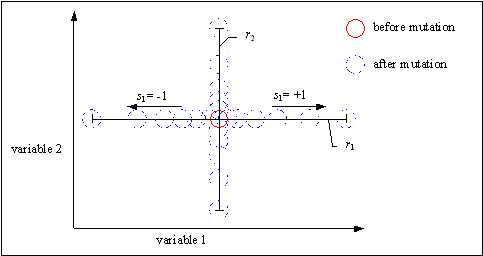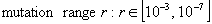GEATbx: Main page  Tutorial  Algorithms  M-functions  Parameter/Options  Example functions  www.geatbx.com

# 5 Mutation

By mutation individuals are randomly altered. These variations (mutation steps) are mostly small. They will be applied to the variables of the individuals with a low probability (mutation probability or mutation rate). Normally, offspring are mutated after being created by recombination.

For the definition of the mutation steps and the mutation rate two approaches exist:

• Both parameters are constant during a whole evolutionary run. Examples are methods for the mutation of real variables, see Section 5.1 and mutation of binary variables, see Section 5.2.
• One or both parameters are adapted according to previous mutations. Examples are the methods for the adaptation of mutation step-sizes known from the area of evolutionary strategies, see Section 5.3.

## 5.1 Real valued mutation

Mutation of real variables means, that randomly created values are added to the variables with a low probability. Thus, the probability of mutating a variable (mutation rate) and the size of the changes for each mutated variable (mutation step) must be defined.

The probability of mutating a variable is inversely proportional to the number of variables (dimensions). The more dimensions one individual has, the smaller is the mutation probability. Different papers reported results for the optimal mutation rate. [MSV93a] writes, that a mutation rate of 1/n (n: number of variables of an individual) produced good results for a wide variety of test functions. That means, that per mutation only one variable per individual is changed/mutated. Thus, the mutation rate is independent of the size of the population.

Similar results are reported in [Bäc93]and [Bäc96] for a binary valued representation. For unimodal functions a mutation rate of 1/n was the best choice. An increase in the mutation rate at the beginning connected with a decrease in the mutation rate to 1/n at the end gave only an insignificant acceleration of the search.

The given recommendations for the mutation rate are only correct for separable functions. However, most real world functions are not fully separable. For these functions no recommendations for the mutation rate can be given. As long as nothing else is known, a mutation rate of 1/n is suggested as well.

The size of the mutation step is usually difficult to choose. The optimal step-size depends on the problem considered and may even vary during the optimization process. It is known, that small steps (small mutation steps) are often successful, especially when the individual is already well adapted. However, larger changes (large mutation steps) can, when successful, produce good results much quicker. Thus, a good mutation operator should often produce small step-sizes with a high probability and large step-sizes with a low probability.

In [MSV93a] and [Müh94] such an operator is proposed (mutation operator of the Breeder Genetic Algorithm):(5-1)

This mutation algorithm is able to generate most points in the hyper-cube defined by the variables of the individual and range of the mutation (the range of mutation is given by the value of the parameter r and the domain of the variables). Most mutated individuals will be generated near the individual before mutation. Only some mutated individuals will be far away from the not mutated individual. That means, the probability of small step-sizes is greater than that of bigger steps. Figure  tries to give an impression of the mutation results of this mutation operator.

Fig. 5-1: Effect of mutation of real variables in two dimensionsThe parameter k (mutation precision) defines indirectly the minimal step-size possible and the distribution of mutation steps inside the mutation range. The smallest relative mutation step-size is 2-k, the largest 20 = 1. Thus, the mutation steps are created inside the area [r, r·2-k] (r: mutation range). With a mutation precision of = 16, the smallest mutation step possible is r·2-16. Thus, when the variables of an individual are so close to the optimum, a further improvement is not possible. This can be circumvented by decreasing the mutation range (restart of the evolutionary run or use of multiple strategies)

Typical values for the parameters of the mutation operator from equation 5-1 are:(5-2)

By changing these parameters very different search strategies can be defined.

## 5.2 Binary mutation

For binary valued individuals mutation means the flipping of variable values, because every variable has only two states. Thus, the size of the mutation step is always 1. For every individual the variable value to change is chosen (mostly uniform at random). Table  shows an example of a binary mutation for an individual with 11 variables, where variable 4 is mutated.

Tab. 5-1: Individual before and after binary mutationAssuming that the above individual decodes a real number in the bounds [1, 10], the effect of the mutation depends on the actual coding. Table  shows the different numbers of the individual before and after mutation for binary/gray and arithmetic/logarithmic coding.

Tab. 5-2: Result of the binary mutationHowever, there is no longer a reason to decode real variables into binary variables. Powerful mutation operators for real variables are available, see the operator in Section 5.1. The advantages of these operators were shown in some publications (for instance [Mic94] and [Dav91]).

## 5.3 Real valued mutation with adaptation of step-sizes

For the mutation of real variables exists the possibility to learn the direction and step-size of successful mutations by adapting these values. These methods are a part of evolutionary strategies ([Sch81] and [Rec94]) and evolutionary programming ([Fdb95]).

Extensions of these methods or new developments were published recently:

• Adaptation of n (number of variables) step-sizes ([OGH93], [OGH94]: ES-algorithm with derandomized mutative step-size control using accumulated information),
• Adaptation of n step-sizes and one direction ([HOG95]: derandomized adaptation of n individual step-sizes and one direction - A II),
• Adaptation of n step-sizes and n directions ([HOG95]: derandomized adaptation of the generating set - A I).

For storing the additional mutation step-sizes and directions additional variables are added to every individual. The number of these additional variables depends on the number of variables n and the method. Each step-size corresponds to one additional variable, one direction to n additional variables. To store n directions n2 additional variables would be needed.

In addition, for the adaptation of n step-sizes n generations with the calculation of multiple individuals each are needed. With n step-sizes and one direction (A II) this adaptation takes 2n generations, for n directions (A I) n2 generations.

When looking at the additional storage space required and the time needed for adaptation it can be derived, that only the first two methods are useful for practical application. Only these methods achieve an adaptation with acceptable expenditure. The adaptation of n directions (A I) is currently only applicable to small problems.

The algorithms for these mutation operators will not be described at this stage. Instead, the interested reader will be directed towards the publications mentioned. An example implementation is contained in [GEATbx]. Some comments important for the practical use of these operators will be given in the following paragraphs.

The mutation operators with step-size adaptation need a different setup for the evolutionary algorithm parameters compared to the other algorithms. The adapting operators employ a small population. Each of these individuals produces a large number of offspring. Only the best of the offspring are reinserted into the population. All parents will be replaced. The selection pressure is 1, because all individuals produce the same number of offspring. No recombination takes place.

Good values for the mentioned parameters are:

• 1 (1-3) individuals per population or subpopulation,
• 5 (3-10) offspring per individual => generation gap = 5,
• the best offspring replace parents => reinsertion rate = 1,
• no selection pressure => SP = 1,
• no recombination.

When these mutation operators were used one problem had to be solved: the initial size of the individual step-sizes. The original publications just give a value of 1. This value is only suitable for a limited number of artificial test functions and when the domain of all variables is equal. For practical use the individual initial step-sizes must be defined depending on the domain of each variable. Further, a problem-specific scaling of the initial step-sizes should be possible. To achieve this the parameter mutation range r can be used, similar to the real valued mutation operator.

Typical values for the mutation range of the adapting mutation operators are:(5-3)

The mutation range determines the initialization of the step-sizes at the beginning of a run only. During the following step-size adaptation the step-sizes are not constrained.

A larger value for the mutation range produces larger initial mutation steps. The offspring are created far away from the parents. Thus, a rough search is performed at the beginning of a run. A small value for the mutation range determines a detailed search at the beginning. Between both extremes the best way to solve the problem at hand must be selected. If the search is too rough, no adaptation takes place. If the initial step sites are too small, the search takes extraordinarily long and/or the search gets stuck in the next small local minimum.

The adapting mutation operators should be especially powerful for the solution of problems with correlated variables. By the adaptation of step-sizes and directions the correlations between variables can be learned. Some problems (for instance the Rosenbrock function - contains a small and curve shaped valley) can be solved very effectively by adapting mutation operators.

The use of the adapting mutation operators is very difficult (or useless), when the objective function contains many minima (extrema) or is noisy.

 GEATbx: Main page  Tutorial  Algorithms  M-functions  Parameter/Options  Example functions  www.geatbx.com

This document is part of version 3.8 of the GEATbx: Genetic and Evolutionary Algorithm Toolbox for use with Matlab - www.geatbx.com.
The Genetic and Evolutionary Algorithm Toolbox is not public domain.